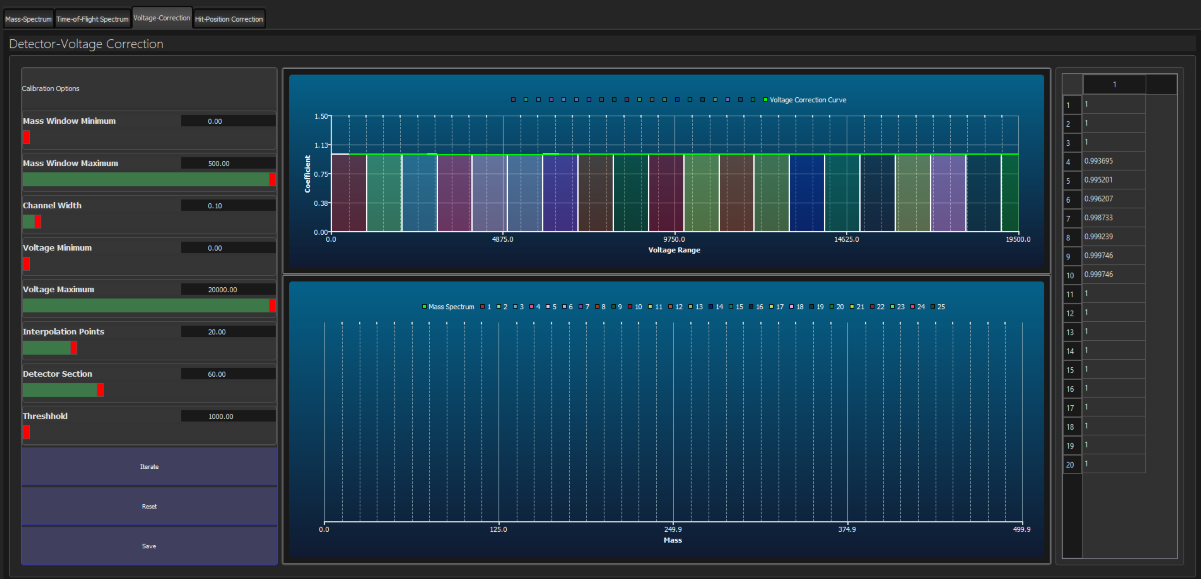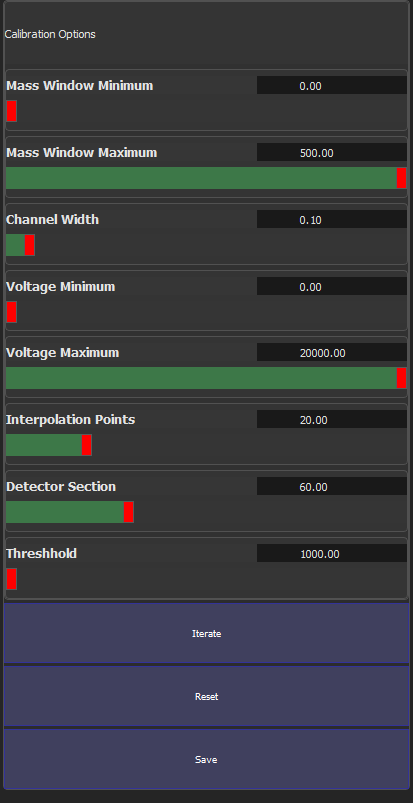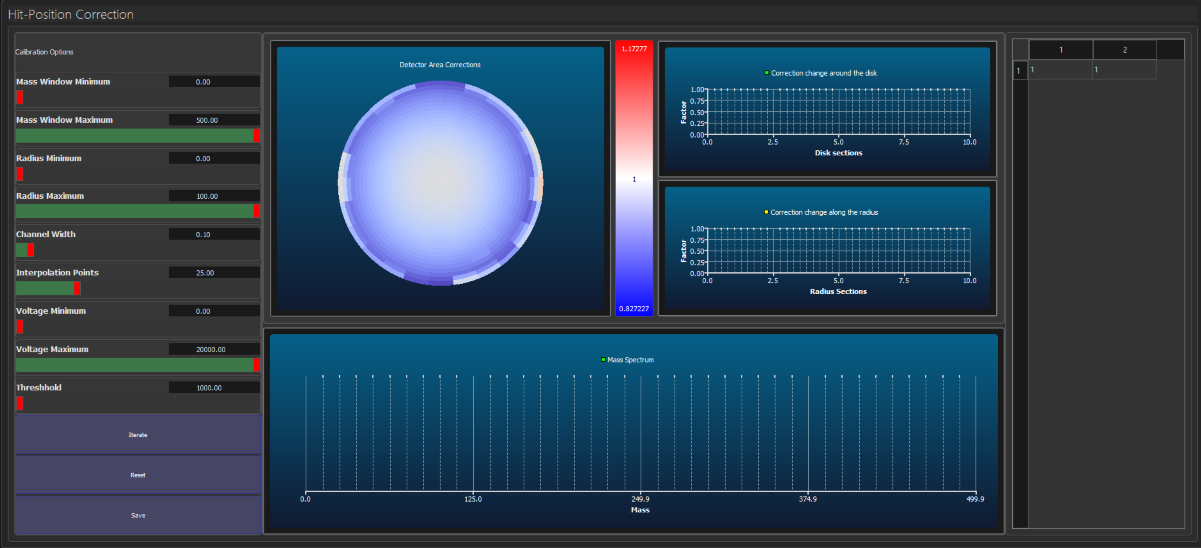Inspico High Resolution Analysis
Dedicated Instruments:  Field Ion Microscopy & Atom Probe Tomography

# Mass Tab - Corrections

The Voltage correction is the third window in the Mass Page. It allows the user to perform a correction on the masses based on the voltage of the events.

The window has 3 main parts. The left side contains parameters needed to calculate the correction and some buttons. The middle part contains 2 graphs, the correction windows and the factors on the top and the Mass Spectrum using the correction at the bottom. The right side contains a table of all the values that you can also modify manually.The calibration options are as follows:• Mass window Minimum and Maximum: you can set the minimum and maximum mass for which you want to calibrate
• Channel width: the width for every Mass Spectrum point. The lower it is the more precise is the mass spectrum
•  Voltage Minimum and Maximum: The maximum and minimum values of voltage for which you want to calibrate
• Interpolation points: Specifies the number of voltage ranges to be created between the minimum and maximum voltage.
• Detector Section: specifies the radius of the detector for which you want to calibrate starting from the center of the detector.
• Threshold: The minimum number of events that a peak should contain for it to be considered.
• Iterate Button: performs one iteration of the voltage calibration. When finished the new factors are calculated and the Mass Spectrum corrected with the calculated factor is displayed. Several iterations may be needed to find the correct corrections
• Reset Button: resets all the correction factors to 1
• Save Button: Saves the current correction factors so that they can be applied to the Mass Spectrum. Use this when you are happy with the factors calculated.

The graphs react when an iteration is performed and update themselves.

The table of values also update automatically after every iteration and also allows for manual input of values.

Hit-Position Correction (HP Correction)

The HP correction assumes a circular Detector and divides it into smaller parts (pie slices and donut levels). Each of these parts (i.e. intersections of slices and levels) has a correction factor which is assigned to its center. Based on the position of every event a correction factor for its mass is calculated using bilinear interpolation of the 4 surrounding detector parts centers.The circular chart is a color coded chart, using the color scale on its right, of all the factors. The two graphs on the right of the color scale react when you click a piece in the detector chart. The green chart display how the factors change along the disk (it is also painted in green in the detector chart. The first column of the left most table displays the exact values. The values start from 0 degrees (north part of the graph) and go all the way around clockwise. In the same way the yellow chart displays the factors along the radius. The second column also displays these values starting from the center of the detector.

If you need to calculate them again then you will need to iterate several times to get the right values.

The controls on the left can help you with that. Mass Window, Radius and Voltage Min/Max filter the data that you want to use for this calibration. Channel width is the width of every mass bar. Interpolation Points is the number of disks and pie slices that are created. Threshold is the lower limit for a peak to be considered a peak.

When you click iterate, a mass spectrum is calculated using the current correction factors. The factor for every part of the detector is calculated so that the highest peak of events in that region si moved to the position of the highest peak in the center of the detector. Every time you iterate the new factors are used to calculate the mass spectrum. In order to save these factor for the current project you have to click the Save Button. The graph on the bottom of the screen shows the current Mass Spectrum after every iteration.